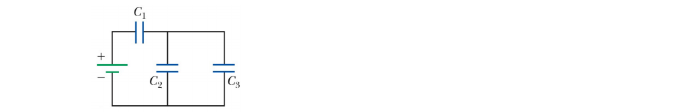# Problem: Three capacitors are connected to a 1 V battery as shown beloe. The capacitances are C1 = 3 F, C2 = 1 F and C3 = 5 F. Rank the capacitors according to the charge they store from largest to smallest. a. Q 3 &gt; Q 1 &gt; Q 2 b. Q 1 &gt; Q 2 = Q 3 c. Q 2 = Q 3 &gt; Q 1 d. Q 1 = Q 2 = Q 3 e. Q 1 &gt; Q 3 &gt; Q 2

###### FREE Expert Solution
93% (355 ratings)
###### Problem Details

Three capacitors are connected to a 1 V battery as shown beloe. The capacitances are C1 = 3 F, C2 = 1 F and C3 = 5 F. Rank the capacitors according to the charge they store from largest to smallest.

a. Q 3 > Q 1 > Q 2

b. Q 1 > Q 2 = Q 3

c. Q 2 = Q 3 > Q 1

d. Q 1 = Q 2 = Q 3

e. Q 1 > Q 3 > Q 2